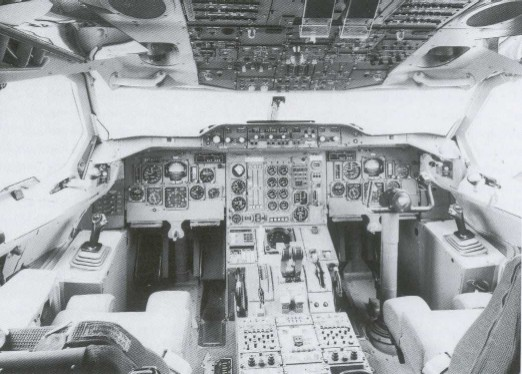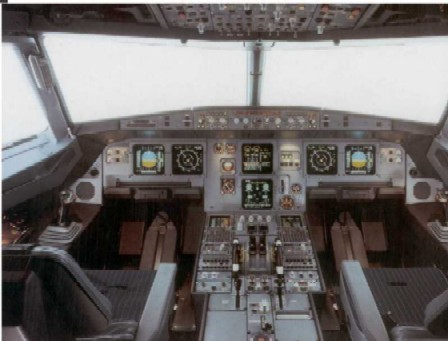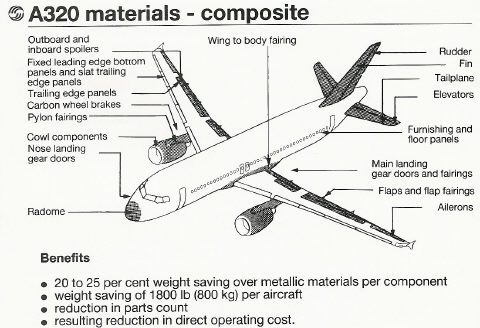AIRBUS TRIVIA

 Notice anything different?  This was the Airbus cockpit (A300) used for testing, utilizing conventional electro- mechanical instruments, but flying with the all new electronic "fly-by-wire" controls ...The end result ...Bigger isn't better ... Depending on the source of information,  the stated wingspan of the A321 is either identical or as much as 7 inches shorter than the A319/320 wing. So, how do you lift an additional 35,000+ pounds with only a 10,000 lb thrust increase (A320 -> A321, 2 engines) ? A significant change to the A321 was the inclusion of double-slotted wing flaps, an extension of the trailing edge to raise the wing area from 1,334 sq ft to 1,377 sq ft, along with an increase in wing camber by altering the trailing edge kink. These changes provide sufficient increased wing area and low-speed lift to maintain the performance characteristics of the A320 wing.

 Plastic?Airbus Inertial Guidance Systems The aircraft knows where it is at all times. It knows this because it knows where it isn't. By subtracting where it is from where it isn't, or where it isn't from where it is (whichever is the greater), it obtains a difference, or deviation. The Inertial Reference System uses deviations to generate error signal commands which instruct the aircraft to move from a position where it is to a position where it isn't, arriving at a position where it wasn't, or now is. Consequently, the position where it is, is now the position where it wasn't; thus, it follows logically that the position where it was is the position where it isn't. In the event that the position where the aircraft now is, is not the position where it wasn't, the Inertial Reference System has acquired a variation. Variations are caused by external factors, the discussions of which are beyond the scope of this report. A variation is the difference between where the aircraft is and where the aircraft wasn't. If the variation is considered to be a factor of significant magnitude, a correction may be applied by the use of the autopilot system. However, use of this correction requires that the aircraft now knows where it was because the variation has modified some of the information which the aircraft has, so it is sure where it isn't. Nevertheless, the aircraft is sure where it isn't (within reason) and it knows where it was. It now subtracts where it should be from where it isn't, where it ought to be from where it wasn't (or vice versa) and integrates the difference with the product of where it shouldn't be and where it was; thus obtaining the difference between its deviation and its variation, which is variable constant called "error".